2. 一元函数数值导数简要介绍¶

:
%matplotlib notebook

import numpy as np
from functools import partial
from pyscf import gto, scf
import matplotlib.pyplot as plt

np.einsum = partial(np.einsum, optimize=["greedy", 1024 ** 3 * 2 / 8])
np.set_printoptions(5, linewidth=150, suppress=True)

2.1. 用于讨论的 Morse 函数¶

$f(x) = \left( 1 - e^{1 - x} \right)^2 - 1$
:
def f(x):
return (1 - np.exp(1 - x))**2 - 1

$f'(x) = \frac{\partial f(x)}{\partial x} = 2 e^{1 - x} \left( 1 - e^{1 - x} \right)$
:
def f_p(x):
return 2 * np.exp(1 - x) * (1 - np.exp(1 - x))

:
xlist = np.arange(0, 5, 0.01)
ylist, yplist = f(xlist), f_p(xlist)

fig, ax = plt.subplots(figsize=(4.5, 3))
ax.plot([0, 5], [0, 0], color="black")
ax.plot(xlist, ylist, label="$f(x)$")
ax.plot(xlist, yplist, label="$f'(x)$")
ax.set_xlim(0, 5), ax.set_ylim(-1, 1)
ax.set_title(r"Plot of $f(x) = x^2$")
ax.set_xlabel(r"$x$")
ax.legend(), ax.grid()
fig.tight_layout()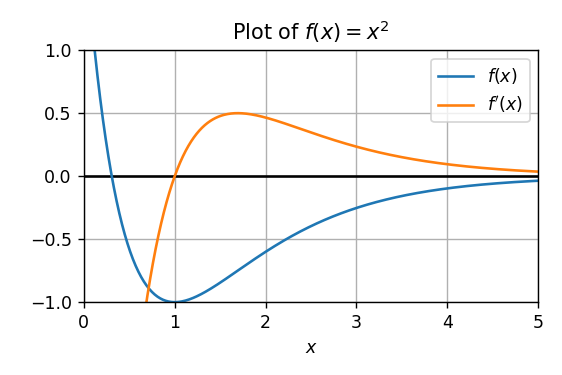2.2. 三点差分一阶数值导数¶

:
xlist = np.arange(2, 4, 0.01)
ylist = f(xlist)
ytlist = f_p(3) * (xlist - 3) + f(3)

fig, ax = plt.subplots(figsize=(4.5, 3))
ax.plot(xlist, ylist, label="$f(x)$")
ax.plot(xlist, ytlist, label="tangent of $(3, f(3))$", linestyle=":")
ax.scatter([2, 3, 4], [f(2), f(3), f(4)], c="C2")
ax.plot([2, 4], [f(2), f(4)], c="C2", label="segment when $h = 1$")
ax.set_xlim(1.8, 4.2), ax.set_ylim(-0.7,-0.0)
ax.set_title(r"Plot of tangent of $(3, f(3))$")
ax.set_xlabel(r"$x$")
ax.legend(), ax.grid()
fig.tight_layout()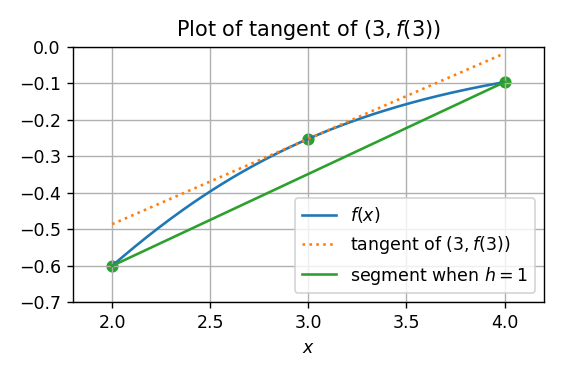$f^\mathrm{d} (x, h) = \frac{f(x + h) - f(x - h)}{2h}$
:
def f_d3(x, h):
return (f(x + h) - f(x - h)) / (2 * h)

$f^\mathrm{d} (3, 1) = \frac{f(4) - f(2)}{4 - 2} \simeq 0.2517$
:
f_d3(3, 1)
:
0.2516641072736052

:
f_p(3)
:
0.23403928869575705

$\lim_{h \rightarrow 0} f^\mathrm{d} (x, h) = f'(x)$

• $$h$$ 较大的情况，数值导数的精度受制于 $$|f^\mathrm{d} (x, h) - f'(x)|$$

• $$h$$ 较小的情况，数值导数的精度受制于计算机本身的精度 (机器精度)；若计算机可以提供相当高的机器精度，那么 $$h$$ 较小时也可以得到良好的数值精度。

:
hlist = np.logspace(-17, 1, 1000)
ylist = np.abs(f_d3(3, hlist) - f_p(3))

fig, ax = plt.subplots(figsize=(4.5, 3))
ax.plot(hlist, ylist)
ax.set_xscale("log"), ax.set_yscale("log")
ax.set_xlabel(r"$h$")
ax.set_title("Log plot of $|f^\mathrm{d} (3, h) - f'(3)|$")
fig.tight_layout()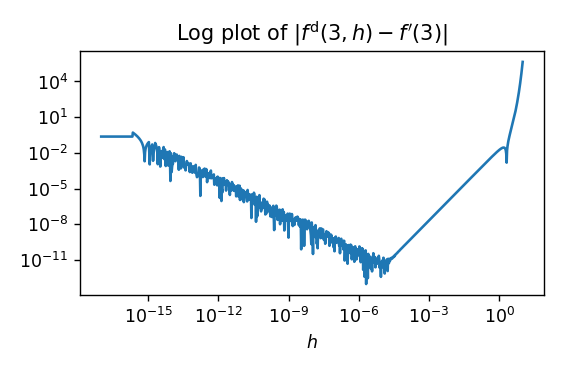2.3. 五点差分一阶数值导数¶

$f^\mathrm{d} (x, h) = \frac{f(x - 2h) - 8 f(x - h) + 8 f(x + h) - f(x + 2h)}{12 h}$
:
def f_d5(x, h):
return (f(x - 2 * h) - 8 * f(x - h) + 8 * f(x + h) - f(x + 2 * h)) / (12 * h)

:
f_p(3), f_d3(3, 1), f_d5(3, 1)
:
(0.23403928869575705, 0.2516641072736052, 0.25524346096060413)

:
f_d3(3, 1e-3) - f_p(3), f_d5(3, 1e-3) - f_p(3)
:
(2.0690889585006644e-08, -1.3128387266192476e-14)

:
hlist = np.logspace(-17, 1, 1000)
y3list = np.abs(f_d3(3, hlist) - f_p(3))
y5list = np.abs(f_d5(3, hlist) - f_p(3))

fig, ax = plt.subplots(figsize=(4.5, 3))
ax.plot(hlist, y3list, label="3-point stencil")
ax.plot(hlist, y5list, label="5-point stencil")
ax.set_xscale("log"), ax.set_yscale("log")
ax.set_xlabel(r"$h$")
ax.set_title("Log plot of $|f^\mathrm{d} (3, h) - f'(3)|$")
ax.legend()
fig.tight_layout()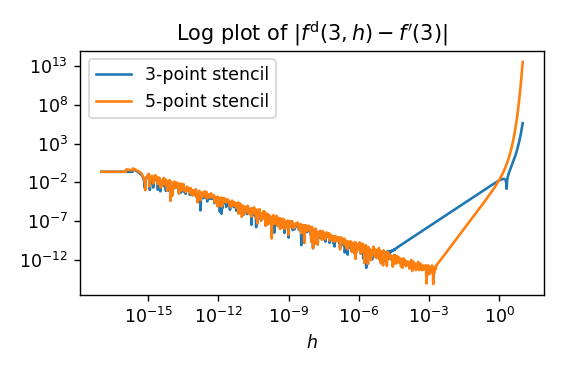• 五点差分的计算代价相对三点差分来说更大。对于一元函数的一阶导数而言，五点差分的代价是三点差分的两倍；但随着函数的未知元数量增大，其计算量还会进一步增大。

• 五点差分在逼近参数 $$h$$ 较大时，未必有更好的逼近表现。

• 五点差分从数学的角度来讲，$$|f^\mathrm{d} (x, h) - f'(x)| = o(h^2)$$；因此函数 $$f^\mathrm{d}(x, h)$$$$f'(x)$$ 的逼近精度随 $$h$$ 越小而平方地更精确 (三点差分是线性地更精确)。因此，若逼近精度不受制于机器精度，那么在 $$h$$ 较小的情况下，五点差分可以更快地逼近到解析导数。

• 但五点差分也更容易受制于机器精度影响，因此 $$h$$ 太小时，误差也未必比三点差分更小。在当前的例子中，$$h$$ 较小的情况下，五点与三点差分的误差近似相等；但对于以后实际的例子，太小的 $$h$$ 很可能带来更为灾难性的结果。

• 希望用较大的 $$h$$ 来逼近时，可以选用五点差分；用较小的 $$h$$ 逼近时，选用三点差分。

• 尽管称为五点差分，但实际上只使用了四个点。这也是由于五点差分的推导所导出的结果。

2.4. 三点差分二阶数值导数¶

$f''(x) = 4 e^{2 - 2x} - 2 e^{1 - x}$
:
def f_pp(x):
return 4 * np.exp(2 - 2 * x) - 2 * np.exp(1 - x)

$f^\mathrm{dd} (x, h) = \frac{f(x - h) - 2 f(x) + f(x + h)}{h^2}$
:
def f_dd(x, h):
return (f(x - h) - 2 * f(x) + f(x + h)) / h**2

$f'{}^\mathrm{d} (x, h) = \frac{f'(x + h) - f'(x - h)}{2h}$
:
def f_pd(x, h):
return (f_p(x + h) - f_p(x - h)) / (2 * h)

:
hlist = np.logspace(-9, 1, 1000)
dlist = np.abs(f_d3(3, hlist) - f_p(3))
ddlist = np.abs(f_dd(3, hlist) - f_pp(3))
pdlist = np.abs(f_pd(3, hlist) - f_pp(3))

fig, ax = plt.subplots(figsize=(4.5, 3))
ax.plot(hlist, dlist, label=r"$f^\mathrm{d} (x, h)$")
ax.plot(hlist, ddlist, label=r"$f^\mathrm{dd} (x, h)$")
ax.plot(hlist, pdlist, label=r"$f'{}^\mathrm{d} (x, h)$")
ax.set_xscale("log"), ax.set_yscale("log")
ax.set_xlabel(r"$h$")
ax.set_title("Error of 3-Point Stencil Derivatives")
ax.legend()
fig.tight_layout()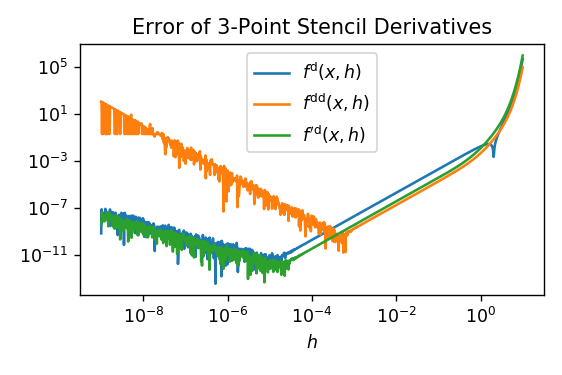• $$f(x)$$ 的两次数值导数 (橙色曲线) 与对 $$f'(x)$$ 的一次数值导数 (绿色曲线) 在稍大的 $$h$$ 值 ($$h > 10^{-3}$$) 时，误差相近；但两次数值导数 (橙色) 应机器精度导致的误差来得更快；

• 一般来说，我们很难判断不同函数的数值导数的误差大小 (拿当前的例子而言，我们不能判断对 $$f(x)$$ 还是对 $$f'(x)$$ 的数值导数误差是哪个更大)，但由于机器精度导致的数值导数振荡的 $$h$$ 值都在 $$10^{-4.5}$$ 左右。